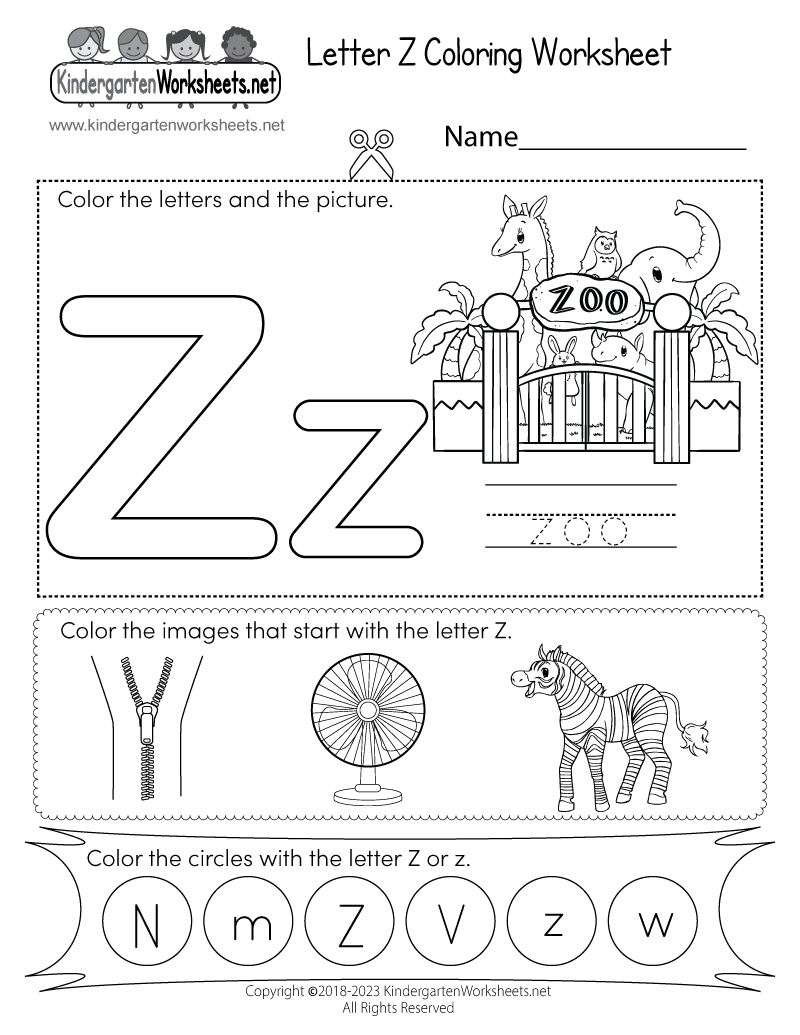## lbartman.com - the pro math teacher

• Subtraction
• Multiplication
• Division
• Decimal
• Time
• Line Number
• Fractions
• Math Word Problem
• Kindergarten
• a + b + c

a - b - c

a x b x c

a : b : c

# Kindergarten English Worksheet

Public on 11 Oct, 2016 by Cyun Lee

###free kindergarten english worksheets printable and online

Name : __________________

Seat Num. : __________________

Date : __________________

### HOW MANY STARS EACH LINE ?

......
......
......
......
......
show printable version !!!hide the show

## RELATED POST

Not Available

## POPULAR

adding fractions with different denominators worksheet

subtraction worksheets to 10

maths free worksheets for grade 3

maths reflection worksheets

maths printable worksheets for grade 2

fractions of numbers worksheets year 3